## Spring Design

Design information for engineers, such as spring calculation formulas,

which are the basis of spring design, can be found here.

### Meaning of Symbols

The symbols used for spring design are shown in Table 1 below. The values of the transverse elastic modulus (G) are based on Table 2.

Table 1. Symbols and units used in calculations

Symbol Meaning of Symbol Unit
a Material width (perpendicular to the central axis) mm
b Material thickness (a = b for square cross-section, parallel to the central axis) mm
D Coil center diameter mm
Di Coil inner diameter mm
Do Coil outer diameter mm
Nt Total number of coils
Na Number of active coils
Hs Solid height mm
Ｈｆ Free length mm
G Transverse elastic modulus N/mm2
P Load applied to the spring N
δ Spring deflection mm
k Spring constant N/mm
τ Torsional correction stress N/mm2
β Stress calculation coefficient
γ Deflection calculation coefficient
Spring index

Table 2. Transverse elastic modulus: G（N/mm2）

Material G Value
Spring steel material 7.85×104
Stainless Steel

SUS304

SUS316

SUS631J1

6.85×104
6.85×104
7.35×104

### Basic Formulas Used for Design

The basic formula for calculating the deflection at the time of compression is calculated using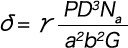The basic formula for the spring constant is calculated by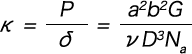The basic formula for stress during compression is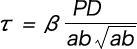The calculation coefficients β and γ differ depending on the spring index (c) and the rectangular shape. Please contact us separately for further details.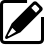Product information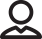Our Proposals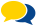Popular questions